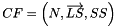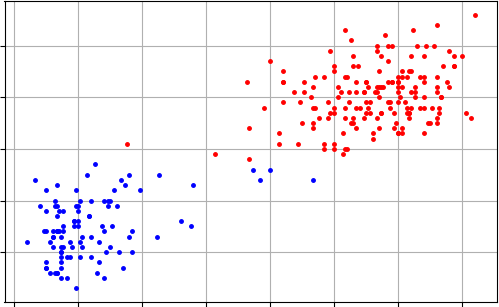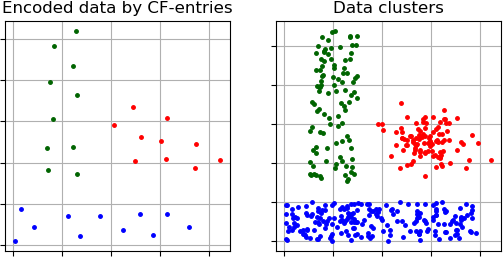pyclustering.cluster.birch.birch Class Reference

Class represents the clustering algorithm BIRCH (Balanced Iterative Reducing and Clustering using Hierarchies). More...

## Public Member Functions

def __init__ (self, data, number_clusters, branching_factor=50, max_node_entries=200, diameter=0.5, type_measurement=measurement_type.CENTROID_EUCLIDEAN_DISTANCE, entry_size_limit=500, diameter_multiplier=1.5, ccore=True)
Constructor of clustering algorithm BIRCH. More...

def process (self)
Performs cluster analysis in line with rules of BIRCH algorithm. More...

def get_clusters (self)
Returns list of allocated clusters, each cluster is represented by a list of indexes where each index corresponds to a point in an input dataset. More...

def get_cf_entries (self)
Returns CF-entries that encodes an input dataset. More...

def get_cf_cluster (self)
Returns list of allocated CF-entry clusters where each cluster is represented by indexes (each index corresponds to CF-entry). More...

def get_cluster_encoding (self)
Returns clustering result representation type that indicate how clusters are encoded. More...

## Detailed Description

Class represents the clustering algorithm BIRCH (Balanced Iterative Reducing and Clustering using Hierarchies).

BIRCH is suitable for large databases. The algorithm incrementally and dynamically clusters incoming multi-dimensional metric data points using the concepts of Clustering Feature and CF tree. A Clustering Feature is a triple summarizing the information that is maintained about a cluster. The Clustering Feature vector is defined as a triple:Example how to extract clusters from 'OldFaithful' sample using BIRCH algorithm:

from pyclustering.cluster.birch import birch
from pyclustering.cluster import cluster_visualizer
from pyclustering.samples.definitions import FAMOUS_SAMPLES
# Sample for cluster analysis (represented by list)
# Create BIRCH algorithm
birch_instance = birch(sample, 2, diameter=3.0)
# Cluster analysis
birch_instance.process()
# Obtain results of clustering
clusters = birch_instance.get_clusters()
# Visualize allocated clusters
visualizer = cluster_visualizer()
visualizer.append_clusters(clusters, sample)
visualizer.show()

Here is the clustering result produced by BIRCH algorithm:Fig. 1. BIRCH clustering - sample 'OldFaithful'.

Methods 'get_cf_entries' and 'get_cf_clusters' can be used to obtain information how does an input data is encoded. Here is an example how the encoding information can be extracted and visualized:

from pyclustering.cluster.birch import birch
from pyclustering.cluster import cluster_visualizer
from pyclustering.samples.definitions import FCPS_SAMPLES
# Sample 'Lsun' for cluster analysis (represented by list of points)
# Create BIRCH algorithm
birch_instance = birch(sample, 3, diameter=0.5)
# Cluster analysis
birch_instance.process()
# Obtain results of clustering
clusters = birch_instance.get_clusters()
# Obtain information how does the 'Lsun' sample is encoded in the CF-tree.
cf_entries = birch_instance.get_cf_entries()
cf_clusters = birch_instance.get_cf_cluster()
cf_centroids = [entry.get_centroid() for entry in cf_entries]
# Visualize allocated clusters
visualizer = cluster_visualizer(2, 2, titles=["Encoded data by CF-entries", "Data clusters"])
visualizer.append_clusters(cf_clusters, cf_centroids, canvas=0)
visualizer.append_clusters(clusters, sample, canvas=1)
visualizer.show()

Here is the clustering result produced by BIRCH algorithm:Fig. 2. CF-tree encoding and BIRCH clustering of 'Lsun' sample.

Definition at line 35 of file birch.py.

## ◆ __init__()

 def pyclustering.cluster.birch.birch.__init__ ( self, data, number_clusters, branching_factor = 50, max_node_entries = 200, diameter = 0.5, type_measurement = measurement_type.CENTROID_EUCLIDEAN_DISTANCE, entry_size_limit = 500, diameter_multiplier = 1.5, ccore = True )

Constructor of clustering algorithm BIRCH.

Parameters
 [in] data (list): An input data represented as a list of points (objects) where each point is be represented by list of coordinates. [in] number_clusters (uint): Amount of clusters that should be allocated. [in] branching_factor (uint): Maximum number of successor that might be contained by each non-leaf node in CF-Tree. [in] max_node_entries (uint): Maximum number of entries that might be contained by each leaf node in CF-Tree. [in] diameter (double): CF-entry diameter that used for CF-Tree construction, it might be increase if 'entry_size_limit' is exceeded. [in] type_measurement (measurement_type): Type measurement used for calculation distance metrics. [in] entry_size_limit (uint): Maximum number of entries that can be stored in CF-Tree, if it is exceeded during creation then the 'diameter' is increased and CF-Tree is rebuilt. [in] diameter_multiplier (double): Multiplier that is used for increasing diameter when 'entry_size_limit' is exceeded. [in] ccore (bool): If True than C++ part of the library is used for processing.

Definition at line 116 of file birch.py.

## ◆ get_cf_cluster()

 def pyclustering.cluster.birch.birch.get_cf_cluster ( self )

Returns list of allocated CF-entry clusters where each cluster is represented by indexes (each index corresponds to CF-entry).

Returns
(list) List of allocated CF-entry clusters.
get_cf_entries

Definition at line 207 of file birch.py.

## ◆ get_cf_entries()

 def pyclustering.cluster.birch.birch.get_cf_entries ( self )

Returns CF-entries that encodes an input dataset.

Returns
(list) CF-entries that encodes an input dataset.
get_cf_cluster

Definition at line 195 of file birch.py.

## ◆ get_cluster_encoding()

 def pyclustering.cluster.birch.birch.get_cluster_encoding ( self )

Returns clustering result representation type that indicate how clusters are encoded.

Returns
(type_encoding) Clustering result representation.
get_clusters()

Definition at line 220 of file birch.py.

## ◆ get_clusters()

 def pyclustering.cluster.birch.birch.get_clusters ( self )

Returns list of allocated clusters, each cluster is represented by a list of indexes where each index corresponds to a point in an input dataset.

Returns
(list) List of allocated clusters.
process()

Definition at line 181 of file birch.py.

## ◆ process()

 def pyclustering.cluster.birch.birch.process ( self )

Performs cluster analysis in line with rules of BIRCH algorithm.

Returns
(birch) Returns itself (BIRCH instance).